# 5G编程聚合网

5G时代下一个聚合的编程学习网

## 1 优化目标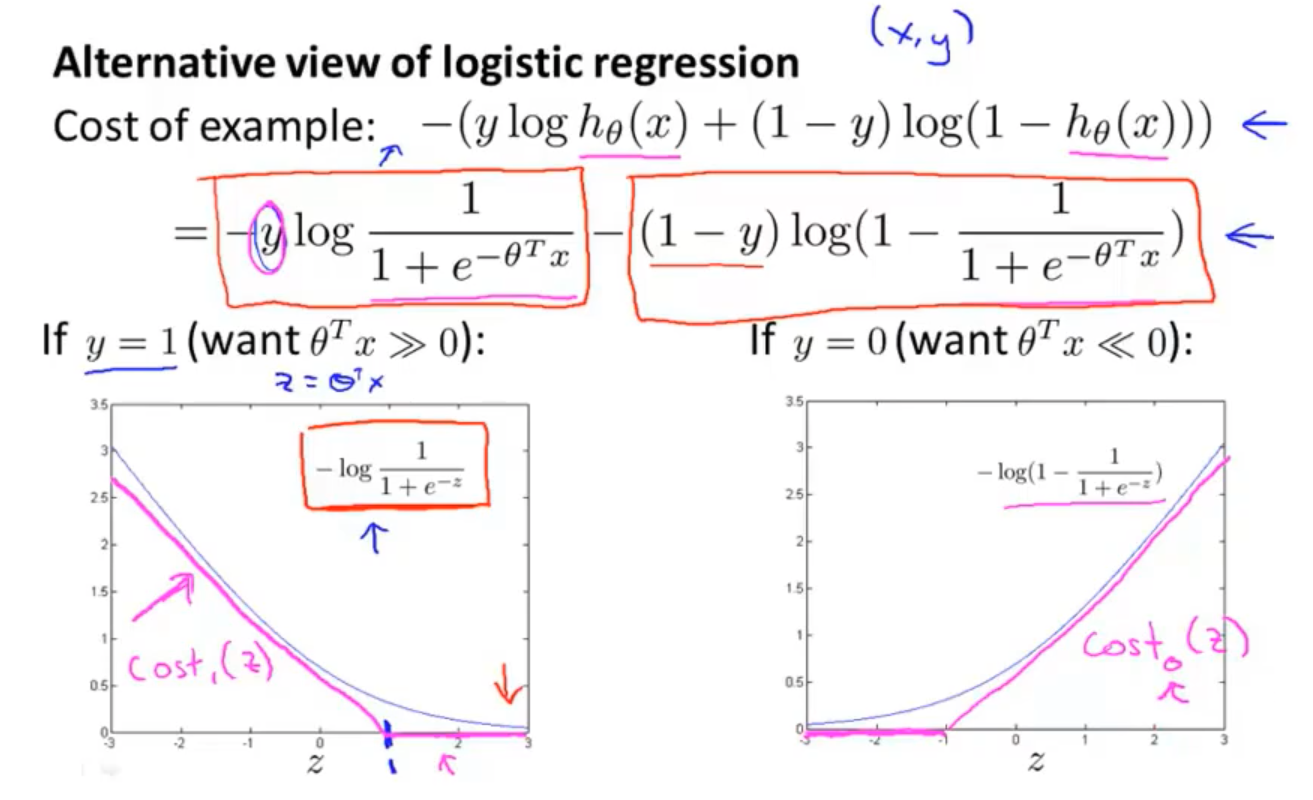• 在逻辑回归中如果有一个 (y=1)的样本，训练的目标则是希望 ({{h}_{ heta }}left( x ight)) 趋近1，对应的 ( heta^Tx) 应当远大于0。

• 相反地，另一个样本即 (y=0)。则希望假设函数的输出值将趋近于0，这对应于 ( heta^Tx) 会远小于0。

• 将逻辑回归的代价函数用分段直线代替如紫色直线所示

[underbrace{frac{1}{m}left[sum_{i=1}^{m} y^{(i)} underbrace{left(-log h_{ heta}left(x^{(i)}ight)ight)}_{cost _{1}left( heta^{ op} x^{(i)}ight)}+left(1-y^{(i)}ight) underbrace{left(left(-log left(1-h_{ heta}left(x^{(i)}ight)ight)ight)ight.}_{cost _{0}left( heta^{ op} x^{(n}ight)}ight]}_{A}+ lambda imes underbrace{frac{1}{2m}sum_{j=1}^{n} heta_{j}^{2}}_{B}
]

A 是训练样本的代价，B 是正则化项。

[min _{ heta} C sum_{i=1}^{m}left[y^{(i)} operatorname{cost}_{1}left( heta^{T} x^{(i)}ight)+left(1-y^{(i)}ight) operatorname{cost}_{0}left( heta^{T} x^{(i)}ight)ight]+frac{1}{2} sum_{i=1}^{n} heta_{j}^{2}
]

[h_{ heta}(x)left{egin{array}{ll}1 & ext { if } heta^{ op} x geqslant 0 \ 0 & ext { othervie }end{array}ight.
]

## 2 大边界分类器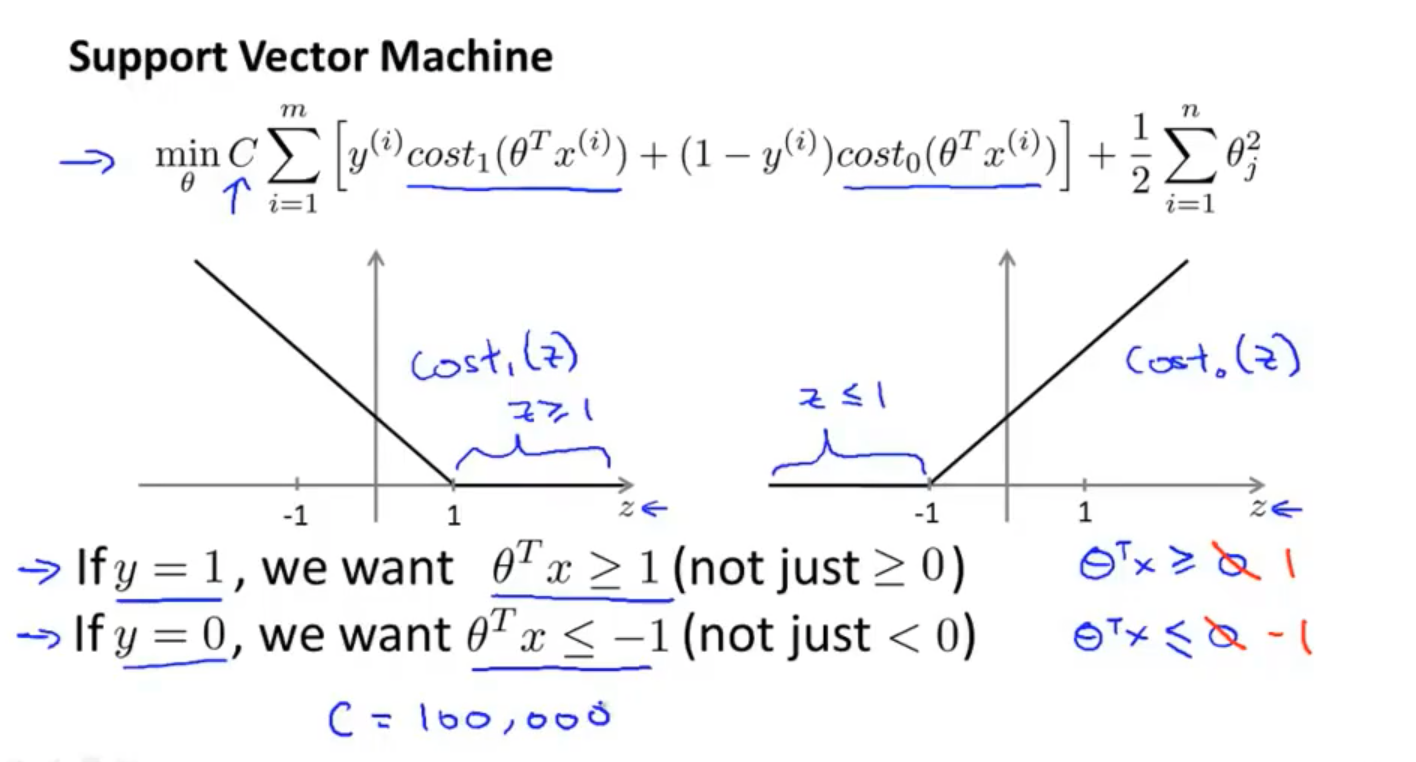• 左边关于 (z) 的代价函数 ({cos}t_1{(z)})，用于正样本，
• 右边关于 (z) 的代价函数 ({cos}t_0{(z)})，用于负样本横轴

• 对于正样本，调整判定边界为 ( heta^Tx>=1)
• 对于负样本调整为 (zleq-1) 的区间里函数值为0。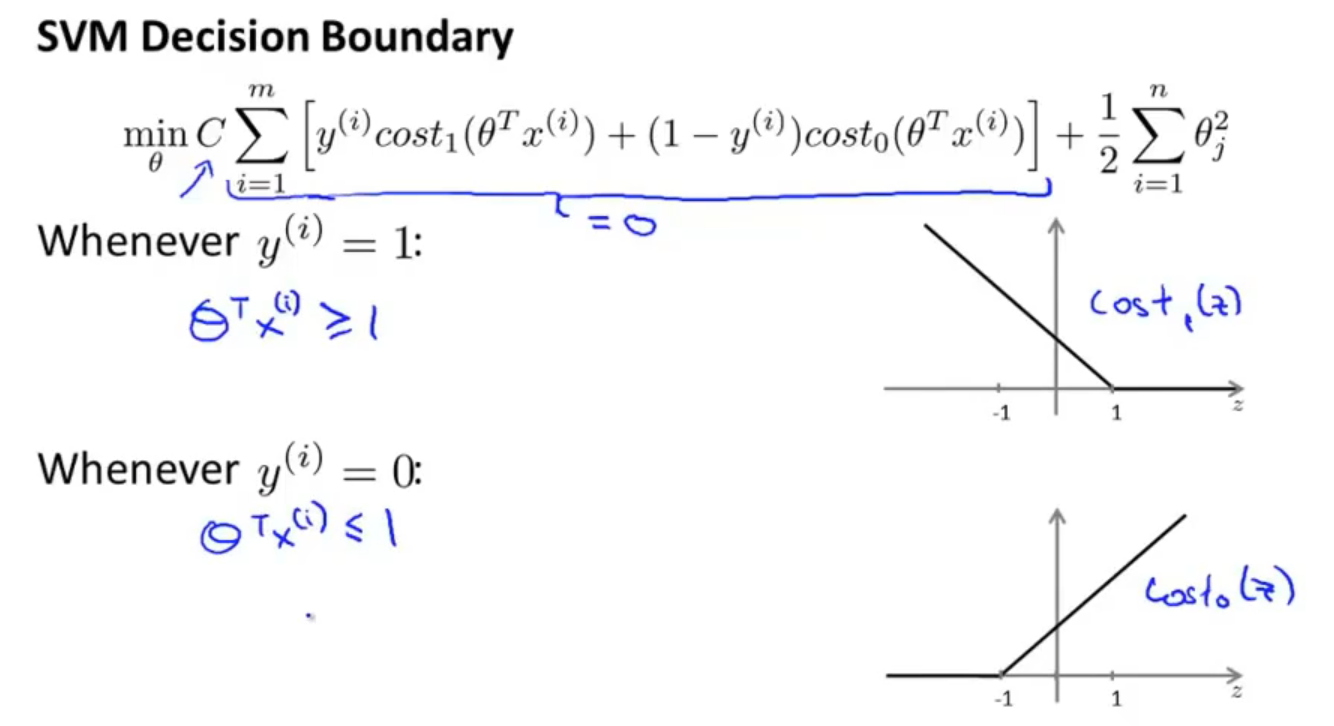[min_limits{ heta}Csum_limits{i=1}^{m}left[y^{(i)}{cos}t_{1}left( heta^{T}x^{(i)}ight)+left(1-y^{(i)}ight){cos}tleft( heta^{T}x^{(i)}ight)ight]+frac{1}{2}sum_limits{i=1}^{n} heta^{2}_{j}
]

• 训练样本标签为 (y=1)，想令第一项为0，需要找到一个 ({{ heta }})，使得( heta^Tx geq 1)

• 训练样本标签为(y=0)，为了使({cos}t_0{(z)}) 函数的值为0，需要 ( heta^Txleq-1)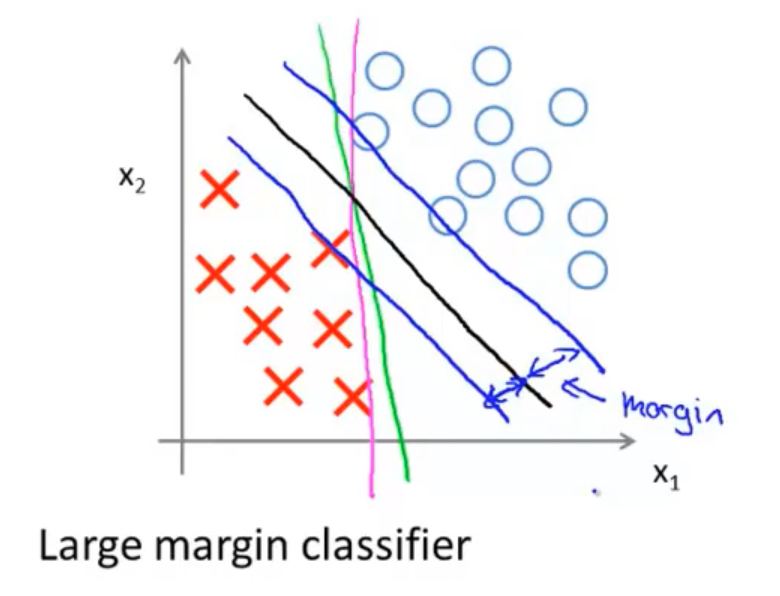[min frac{1}{2} sum_{j=1}^{n} heta_{j}^{2} ext { s.t }left{egin{array}{c} heta^{T} x^{(i)} geq 1 ext { if } y^{(i)}=1 \ heta^{T} x^{(i)} leq-1 ext { if } y^{(i)}=0end{array}ight)
]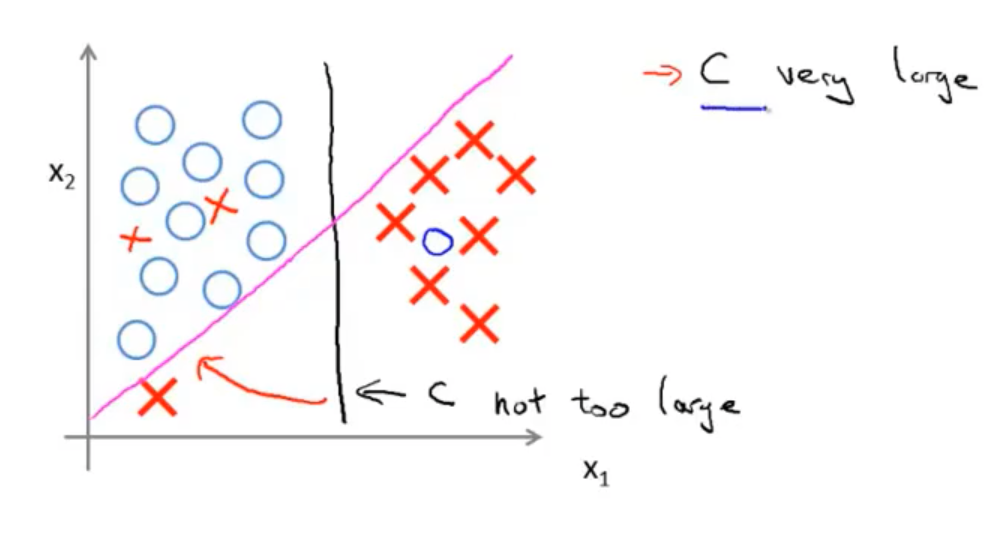(C)不是非常非常大的时候，它可以忽略掉一些异常点的影响，得到更好的决策界。甚至当你的数据不是线性可分的时候，支持向量机也可以给出好的结果。

(C=1/lambda)

• (C) 较大时，相当于 (lambda) 较小，可能会导致过拟合，高方差。

• (C) 较小时，相当于(lambda)较大，可能会导致低拟合，高偏差。

## 3 大边界分类数学原理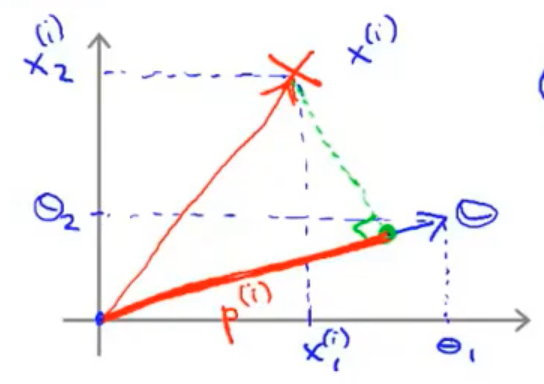(θ^Tx^{(i)}) 等于(x^{(i)})( heta) 向量方向上的投影 (p^{(i)})(|| heta||)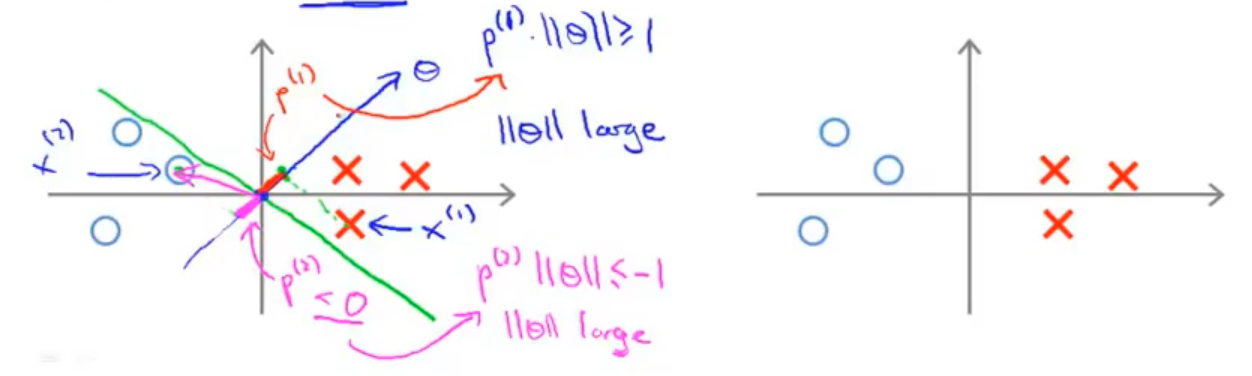(p^{(i)}) 都是非常小的数，因此优化目标函数时，对正样本而言，需满足 (p^{(i)}cdot{left| heta ight|}>=1), 如果 (p^{(i)}) 非常小, 意味 ({{ heta }}) 范数要非常大.对于负样本同理。但优化目标是 (min.frac{1}{2}left| heta ight|^2)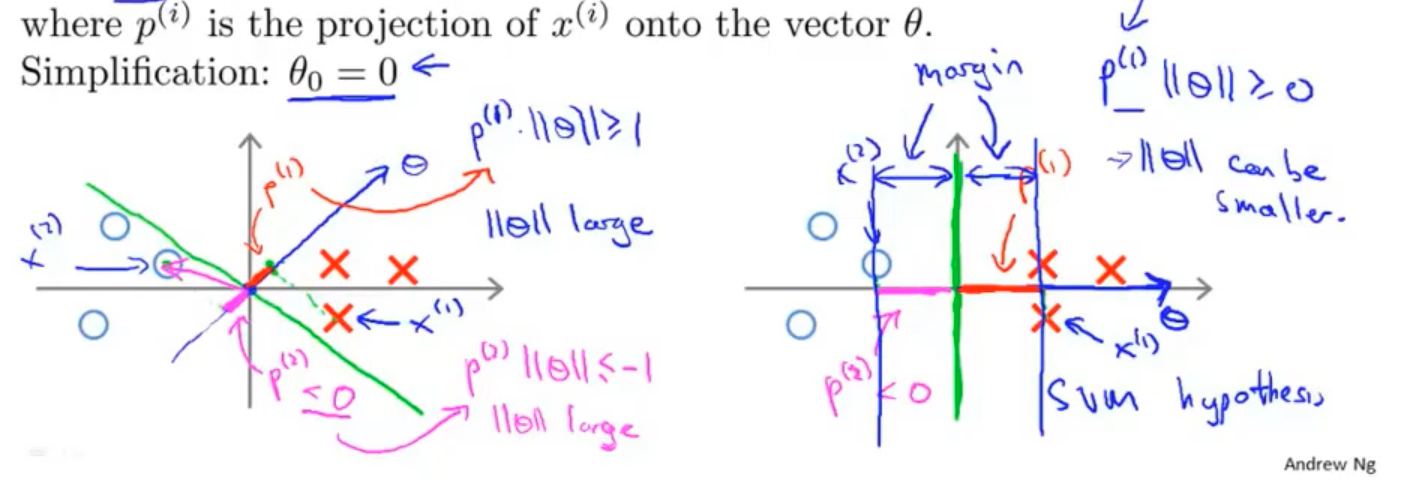## 4 高斯核函数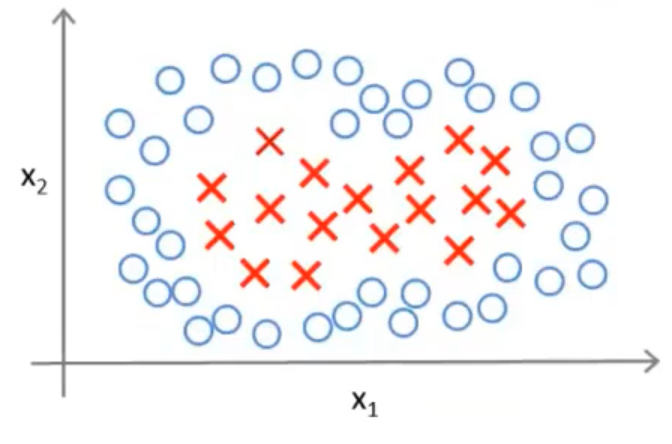[{{f}_{1}}={{x}_{1}},{{f}_{2}}={{x}_{2}},{{f}_{3}}={{x}_{1}}{{x}_{2}},{{f}_{4}}=x_{1}^{2},{{f}_{5}}=x_{2}^{2}，cdots
]

[h_θ(x)={{ heta }_{1}}f_1+{{ heta }_{2}}f_2+…+{{ heta }_{n}}f_n
]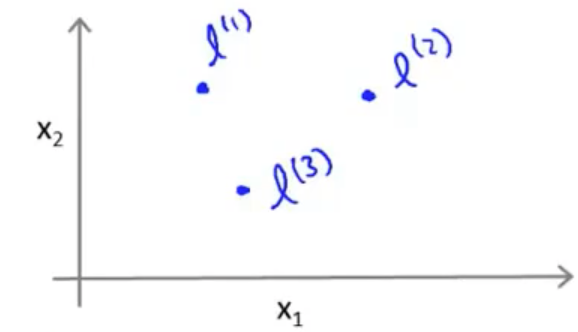[{{f}_{1}}=kappa(x,{{l}^{(1)}})=expleft({-frac{{{left| x-{{l}^{(1)}} ight|}^{2}}}{2{{sigma }^{2}}}}ight)
]

[{{left| x-{{l}^{(1)}} ight|}^{2}}=sum{_{j=1}^{n}}{{({{x}_{j}}-l_{j}^{(1)})}^{2}}
]

(kappa(x,{{l}^{(1)}})) 就是核函数，这里是一个高斯核函数(Gaussian Kernel)。 注：这个函数与正态分布没什么实际上的关系，只是看上去像而已。

• 如果一个训练样本 (x) 与地标 (l) 之间的距离近似于 0，则新特征 (f) 近似于(e^{-0}=1)

• 如果训练样本 (x) 与地标 (l) 之间距离较远，则 (f) 近似于 (e^{-(INF)}approx0)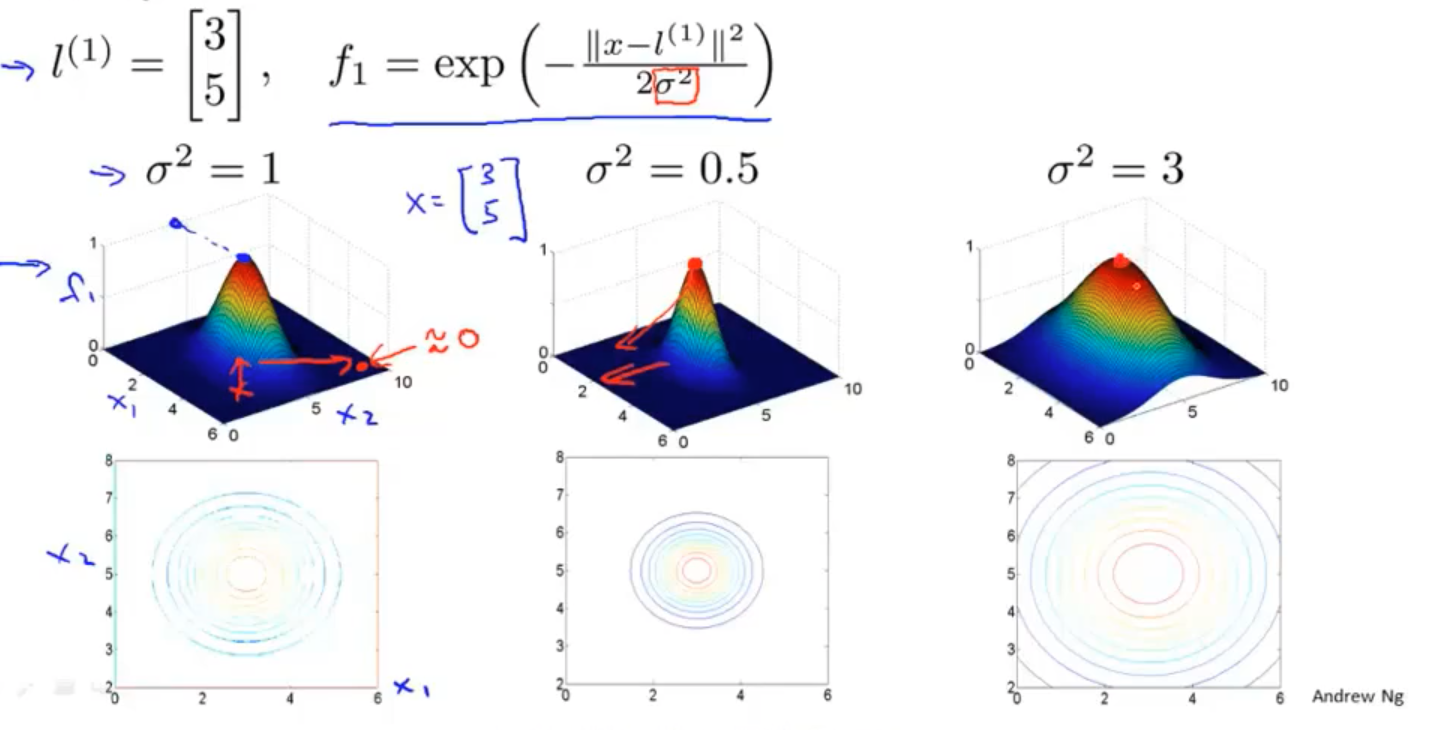• 下图中，当样本处于粉色点位置处，其离 (l^{(1)}) 更近，离 (l^{(2)})(l^{(3)}) 较远，因此 (f_1) 接近1，而(f_2), (f_3) 接近0。(h_θ(x)=θ_0+θ_1f_1+θ_2f_2+θ_1f_3>0)，因此预测(y=1)
• 同理，对于离 (l^{(2)}) 较近的绿色点，也预测 (y=1)，但是对于蓝绿色的点，因为其离三个地标都较远，预测 (y=0)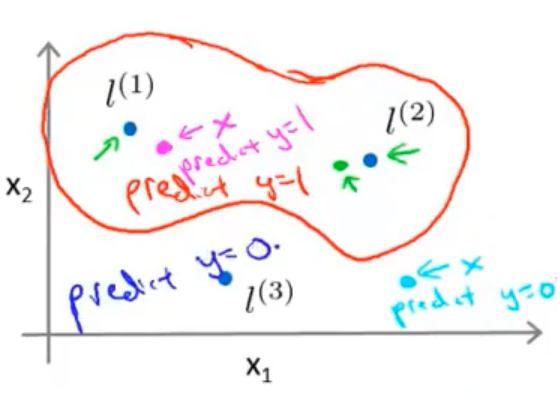## 5 地标选择

[l^{(1)}=x^{(1)},l^{(2)}=x^{(2)},…..,l^{(m)}=x^{(m)}
]

[f^{(i)}=left[egin{array}{c}f_{0}^{(i)}=1 \ f_{1}^{(i)}=operatorname{kappa}left(x^{(i)}, l^{(1)}ight) \ f_{2}^{(i)}=operatorname{kappa}left(x^{(i)}, l^{(2)}ight) \ f_{i}^{(i)}=operatorname{kappa}left(x^{(i)}, l^{(i)}ight)=e^{0}=1 \ vdots \ f_{m}^{(i)}=operatorname{kappa}left(x^{(i)}, l^{(m)}ight)end{array}ight]
]

[h_{ heta}(f)left{egin{array}{ll}1 & ext { if } heta^{ op} f geqslant 0 \ 0 & ext { othervie }end{array}ight.
]

[sum{_{j=1}^{n=m}}~~ heta _{j}^{2}={{ heta}^{T}} heta
]

[min. Csumlimits_{i=1}^{m}{[{{y}^{(i)}}cos {{t}_{1}}}( {{ heta }^{T}}{{f}^{(i)}})+(1-{{y}^{(i)}})cos {{t}_{0}}( {{ heta }^{T}}{{f}^{(i)}})]+frac{1}{2}sumlimits_{j=1}^{n=m}{ heta _{j}^{2}}
]

• (C) 较大时，相当于(lambda)较小，可能会导致过拟合，高方差；

• (C) 较小时，相当于(lambda)较大，可能会导致低拟合，高偏差；

• (sigma) 较大时，可能会导致低方差，高偏差；

• (sigma) 较小时，可能会导致低偏差，高方差。

## 6 使用 SVM

• 多项式核函数（Polynomial Kernel）
[kappaleft(oldsymbol{x}_{i}, oldsymbol{x}_{j}ight)=left(oldsymbol{x}_{i}^{mathbf{T}} oldsymbol{x}_{j}ight)^{d}，dgeq 1 ext{为多项式的次数}
]

• 卡方核函数（ chi-square kernel, (chi^{2}) kernel
[kappa(mathbf{x}, mathbf{y})=1-sum_{i=1}^{n} frac{left(x_{i}-y_{i}ight)^{2}}{frac{1}{2}left(x_{i}+y_{i}ight)}, ext { for } mathbf{x},quad mathbf{y} in mathbb{R}^{n}
]

• 拉普拉斯核函数
]

• Sigmoid 核函数
[kappaleft(oldsymbol{x}_{i}, oldsymbol{x}_{j}ight)= anh left(eta oldsymbol{x}_{i}^{mathrm{T}} oldsymbol{x}_{j}+ hetaight) quad anh ext { 为双曲正切函数, } eta>0, heta<0
]

(n) 为特征数，(m) 为样本数。

(1) 相较于 (m)(n)要大许多，即训练集数据量不够支持我们训练一个复杂的非线性模型，选用逻辑回归模型或者不带核函数的支持向量机。

(2) 如果 (n) 较小，而且 (m) 大小中等，例如(n)在 1-1000 之间，而(m)在10-10000之间，使用高斯核函数的支持向量机。

(3) 如果 (n) 较小，而 (m) 较大，例如 (n) 在 1-1000 之间，而 (m) 大于 50000，则使用支持向量机会非常慢，解决方案是创造、增加更多的特征，然后使用逻辑回归或不带核函数的支持向量机。